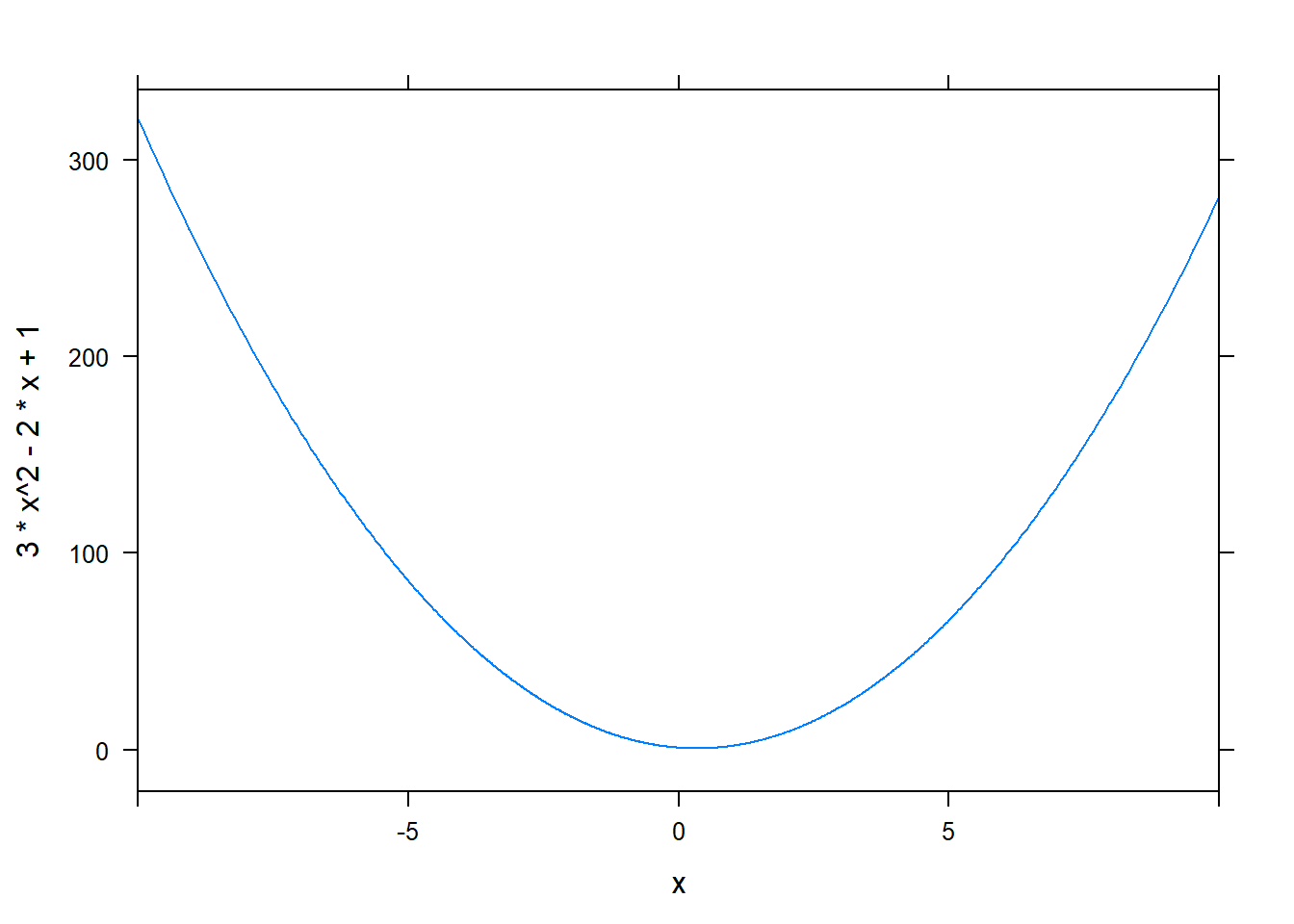Want to share your content on R-bloggers? click here if you have a blog, or here if you don't.

## The basics

This is a compilation of basic codes for doing elementary mathematics (college algebra, calculus)

The best software for doing mathematics may be Mathematica, Maple, MatLab, Sage and other software. However, you can also do some math using R with the convenience of RStudio and R Markdown.

# install.packages("mosaic", "mosaicCalc")

require(mosaic)
require(mosaicCalc)

## Given g(x)=3*x^2 -2*x +1, find g(2) #base R, that is,no extra package is used.
g <- function(x){3*x^2 -2*x +1}
g(2)
##  9

## Given g(x)=3*x^2 -2*x +1, find g(2) ##mosaic
g<-makeFun(3*x^2 -2*x +1 ~ x)
g(2)
##  9

##Plot the function y=3x^2-x-2 on the interval [-10, 10] ##base R
curve(3*x^2 -2*x +1, -10, 10)## Plot the function y=3x^2-x-2 on the interval [-10, 10] ##moasaic
plotFun(3*x^2 -2*x +1 ~x, x.lim=range(-10,10) )## Plot the function y=3x^2-x-2 on the interval [-10, 10]. ##ggplot2
library("ggplot2")
eq = function(x){3*x^2 -2*x +1}
g<-ggplot(data.frame(x=c(-10, 10)), aes(x=x))
g<-g+ stat_function(fun=eq)
g## Solve the equation x^2 +x -6=0 for x.
findZeros(x^2 +x -6 ~ x,x.lim=range(-Inf, Inf))
## x
## 1 -3
## 2 2

## Given F(x)= 3*x^2 - 2*x +1, find F'(2) (derivative of F at x=2)
g<- D(3*x^2 -2*x + 1 ~ x)
g(2)
##  10

## Evaluate the definite integral \int_{-1}^{2}(x^2-2x+1)dx
F<-antiD(x^2-2*x+1 ~ x)
F(2)-F(1)
##  0.3333333

(DRAFT=TRUE; will be updated)